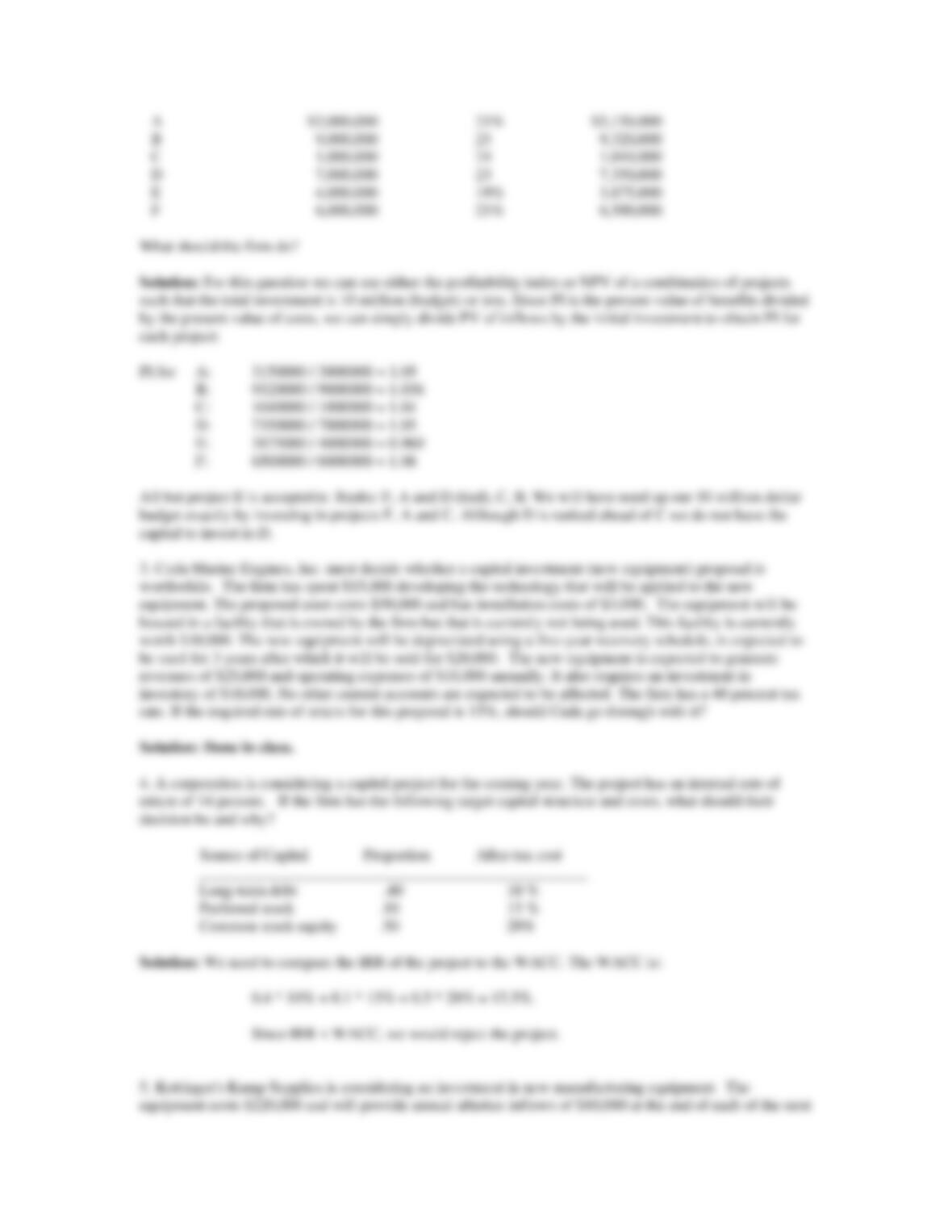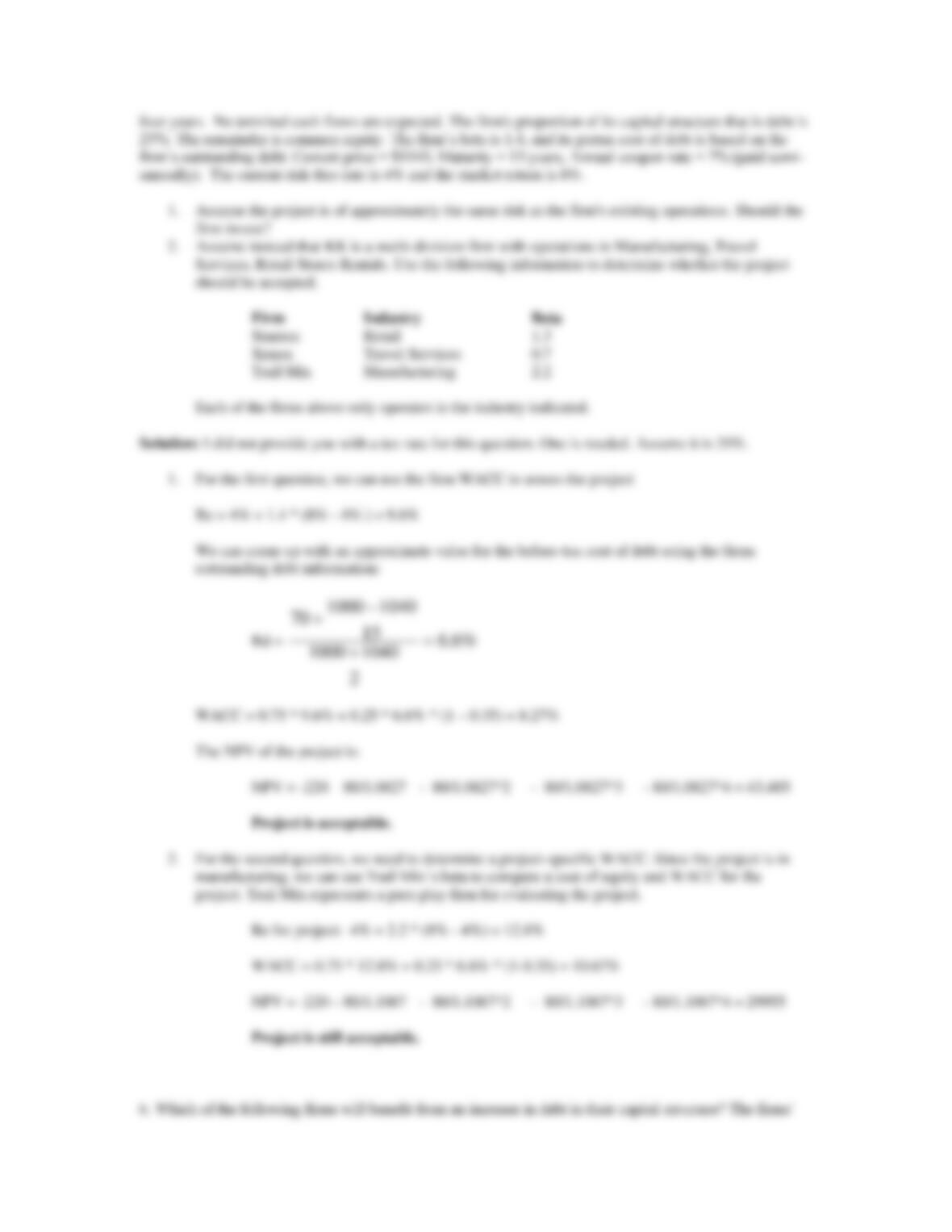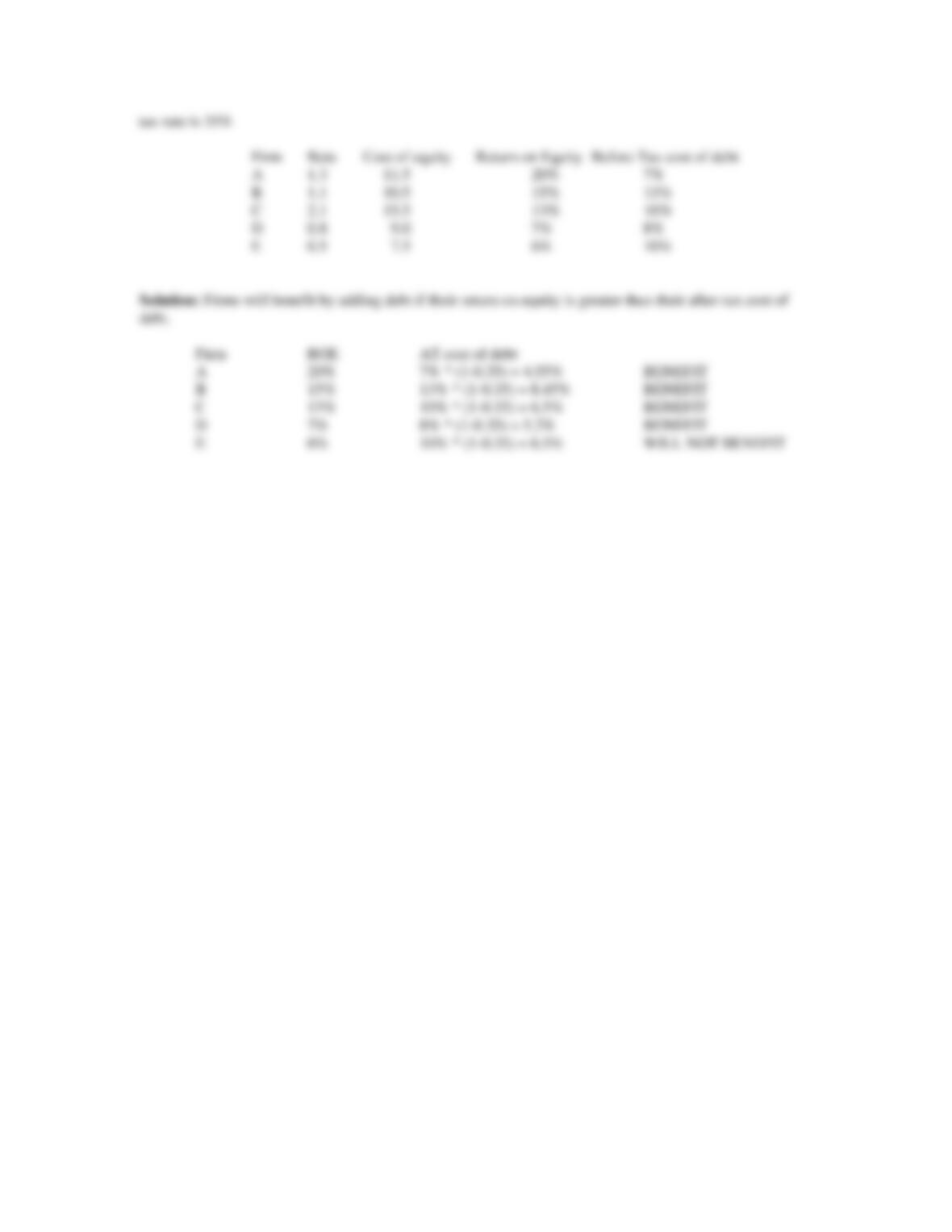Type
Essay
Pages
5 pages
Word Count
1035 words
School
Valparaiso University
Course Code
Finance

# Finance

November 22, 2020
BA 340
Final review questions solutions
1. Degnan Dance Company, Inc., a manufacturer of dance and exercise apparel, is considering replacing an
existing piece of equipment with a more sophisticated machine. The following information is given.
Existing Machine Proposed Machine
_________________________________________________________________
Cost = \$100,000 Cost = \$150,000
Purchased 2 years ago Installation = \$20,000
Depreciation using MACRS over Depreciation--the MACRS
a 5-year recover schedule 5-year recovery schedule will be used.
Current market value = \$105,000
Five year usable life remaining Five year usable life expected
Earnings Before Depreciation and Taxes
Existing Machine Proposed Machine
________________________________________________________________
Year 1 \$160,000 Year 1 \$170,000
2 150,000 2 170,000
3 140,000 3 170,000
4 140,000 4 170,000
5 140,000 5 170,000
The proposed machine would be sold at book value at the end of the fifth year. The existing machine would
be discarded after its remaining useful life if it was maintained. No working capital investment is required.
The firm’s cost of capital is 10% and its tax rate is 40%. Should the new machine be purchased?
Solution: Since we are evaluating just one project, we can use NPV.
The required rate of return is 10%
Incremental cash flows:
Proposed machine:
Initial cash flows: Purchase price + installation = \$170,000
Operating cash flows:
Using a 5-yr MACRS schedule, depreciation for the proposed machine for 5 years is:
Yr. 1: 170000 * 0.2 = 34000
2: 170000 * 0.32 = 54400
3: 32640
4: 19580
5: 19580
OCFs: Yr 1 2 3 4 5
EBDepr 170000 170000 170000 170000 170000
- Depr 34000 54400 32640 19580 19580
EBIT 136000 115600 137360 150420 150420
-taxes 54400 46240 54948 60168 60168
+Depr 34000 54400 32640 19580 19580
OCF 115600 123760 115052 109832 109832
Terminal cash flow:
Sale of proposed machine (at book value):
Book value = 170000 34000 54400 32640 19580 19580 = 9800
Existing machine:
Sale of existing machine (included in initial cash flows):
Book value = 100000 (100000 * 0.2) (100000 * 0.32) = 48000
Cash flow from sale: 105000 (105000 48000) * 0.4 = 82200
Operating cash flows lost:
The existing machine is to be used for 5 more years:
Depreciation:
Yr. 1: 100000 * 0.192 = 19200
2: 100000 * 0.1152 = 11520
3: 100000 * 0.1152 = 11520
4: 100000 * 0.0576 = 5760
5: 0
OCFs: Yr 1 2 3 4 5
EBDepr 160000 150000 140000 140000 140000
- Depr 19200 11520 11520 5760 0
EBIT 140800 138480 128480 134240 140000
-taxes 56320 55392 51392 53696 56000
+Depr 19200 11520 11520 5760 0
OCF 103680 94608 88608 86304 84000
NPV:
(-170000 + 82200) + (115600 103680)/1.1
+ (123760 94608)/1.1^2 + (115052 88608) / 1.1^3 + (109832 86304)/1.1^4
+ (109832 + 9800 84000) / 1.1^5 = 5191 ACCEPT.
2. Galaxy Satellite Co. is attempting to select the best group of independent projects competing for the
firm's fixed capital budget of \$10,000,000. All four projects have conventional cash flows. The firm’s cost
of capital is 20%. A summary of key data about the proposed projects follows.
PV of Inflows
Project Initial Investment IRR at 20%
------- ------------------ --- -------------A \$3,000,000 21% \$3,150,000
B 9,000,000 25 9,320,000
C 1,000,000 24 1,040,000
D 7,000,000 23 7,350,000
E 4,000,000 19% 3,875,000
F 6,000,000 21% 6,500,000
What should the firm do?
Solution: For this question we can use either the profitability index or NPV of a combination of projects
such that the total investment is 10 million (budget) or less. Since PI is the present value of benefits divided
by the present value of costs, we can simply divide PV of inflows by the initial investment to obtain PI for
each project:
PI for A: 3150000 / 3000000 = 1.05
B: 9320000 / 9000000 = 1.036
C: 1040000 / 1000000 = 1.04
D: 7350000 / 7000000 = 1.05
E: 3875000 / 4000000 = 0.969
F: 6500000 / 6000000 = 1.08